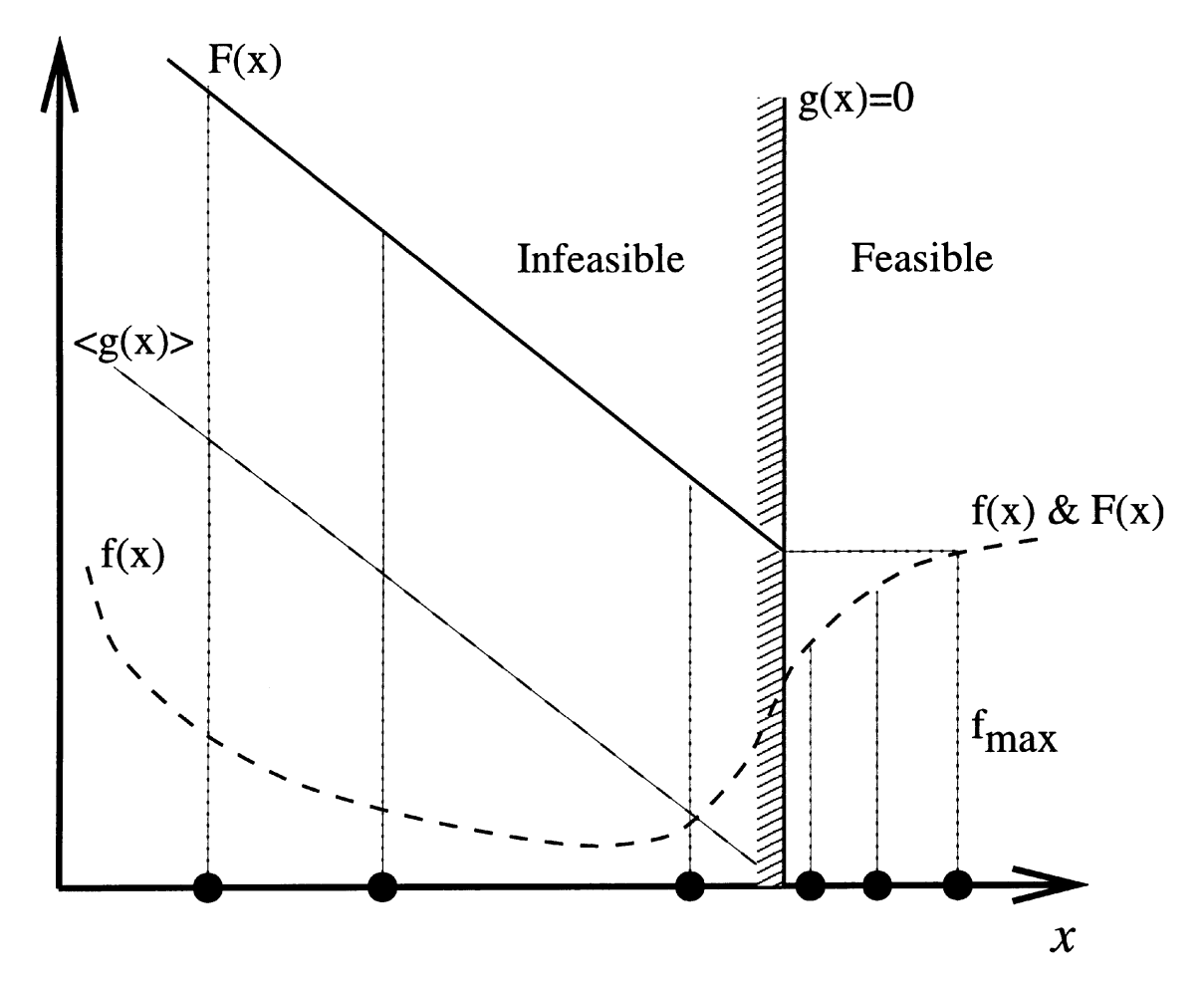# Evolutionary Single-objective Optimization

COIN lab also addresses fundamental aspects of single-objective evolutionary algorithms and attempts to develop efficient methodologies. Some of Prof. Deb’s earlier studies are now used as default methodologies. The penalty parameter-less constraint handling approach (CMAME, 2000) is one such contribution. The simulated binary recombination (SBX) operator proposed in 1995 is another real-parameter recombination operator which is routinely used in EC studies and has also been adopted in commercial softwares. Similarly, polynomial mutation operator (suggested in Deb’s (2001) book) is another commonly-used EC operator. Currently, COIN lab is investigating a non-uniform mapping binary-coded genetic algorithm and its extension for a better application. Another study on creating an efficient initial population for limited budget application is interesting.## Parameter-less Constraint Handling

Constraints are inevitable in practice. However, there has been a lukewarm interest shown by EMO researchers in making their algorithms applied to constrained problems. Research at COIN lab has always focused on unconstrained and constrained problems alike. In 2000, we developed a parameter-less constraint handling approach, which is now known as 'Deb's Parameter-less Approach'. Without using any parameter the methodology compares two solutions by emphasizing feasible over infeasible, less violated infeasible, and better objective-based feasible solutions. The idea can be implemented wither by changing the binary tournament selection or by defining a Fitness function using objective and constraint violations. The idea has also been extended for multi-objective optmization by redefining domination principle using 'constrained domination principle'.

#### References

Deb, K. (2000). An eﬃcient constraint handling method for genetic algorithms. Computer Methods in Applied Mechanics and Engineering, 186, 311–338.

Deb, K., Pratap. A, Agarwal, S., and Meyarivan, T. (2002). A fast and elitist multiobjective genetic algorithm: NSGA-II. IEEE Transaction on Evolutionary Computation, 6(2), 181–197.

## Real-parameter Recombination Operator - Simulated Binary Crossover

While recombining two real-parameter vectors to create one or more real-parameter vectors, EC researchers have proposed ad-hoc procedures by restricting offsprings vectors to be picked uniformly from the region enclosed (or extended) by two parent vectors. In 1995, we have shown that if real variables are coded in long binary strings, the resulting single-point crossover operators will create offsprings which are not uniformly distributed, rather has a biased distribution closer to the parent vectors. This is due to the fact that there are more crossovers happen at the least significant bits resulting in near parent points. Based on this observation, we have proposed Simulated Binary Crossover (SBX) in which a non-dimensionalized probability distribution is used to create offsprings near parents variable wise. The distribution index eta_c controls the relative spread of offspring from parent. Higher eta_c means lesser spread. The idea was later extended to have Parent-centric crossover (PCX) operated vector-wise.

#### References

Deb, K. and Agrawal, R. B. (1995). Simulated binary crossover for continuous search space. Complex Systems, 9, 115–148.

Deb, K. and Kumar, A. (1995). Real-coded genetic algorithms with simulated binary crossover: Studies on multimodal and multiobjective problems. Complex Systems, 9(6), 431–454.

Deb, K. and Beyer, H.-G. (2001). Self-adaptive genetic algorithms with simulated binary crossover. Evolutionary Computation Journal, 9(2), 197–221.

Deb, K., Joshi, D., and Anand, A. (2002). Real-coded evolutionary algorithms with parentcentric recombination. Proceedings of the Congress on Evolutionary Computation (CEC2002). (Honolulu, USA). (pp. 61–66).

## Real-parameter Mutation Operator - Polynomial Mutation

Real-parameter mutation means a perturbation of an existing parent vector to a neighboring vector. Gaussian probabilities are often used for such purposes with a user-defined variance. In polynomial mutation, a polynomial probability distribution was proposed which is similar in principle to Gaussian distribution. The distribution index eta_m controls the spread of mutated point from parent. Higher eta_m produces smaller spread. A comparison of polynomial mutation with Gaussian mutation can be found at the second reference given below.

#### References

Deb, K. (2001). Multi-objective optimization using evolutionary algorithms. Chichester, London: Wiley.

Deb, K. and Deb, D. (2014). Analysing mutation schemes for real-parameter genetic algorithms. Int. J. Artiﬁcial Intelligence and Soft Computing, 4(1), Inderscience Enterprises Ltd., 1–28 (DOI: 10.1504/IJAISC.2014.059280)